# How many bags of concrete do i need for a 10' x 10' slab?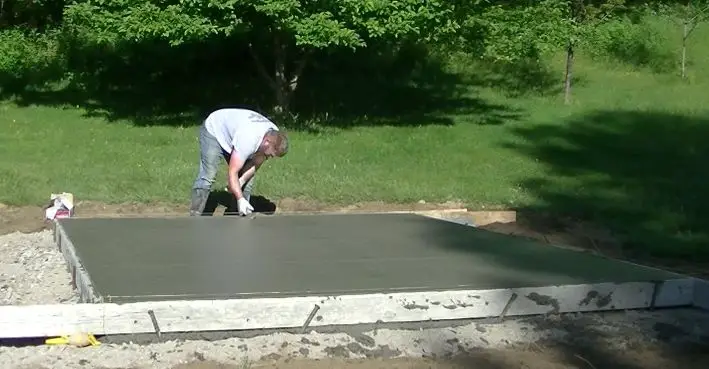The amount of concrete I used for this 10' x 10' slab was 2 yards.

First, here's how many yards of concrete you need for a 10 x 10 slab based on how thick it is.

The amount of concrete you need for a 10 x 10 slab that's 3 inches thick is 1 cubic yard.

• A 10 x 10 slab that's 4 inches thick needs 1.25 cubic yards or (56) 80lb bags of concrete
• A 10 x 10 slab that's 5 inches thick needs 1.6 cubic yards or (72) 80lb bags of concrete
• A 10 x 10 slab that's 6 inches thick needs 1.85 cubic yards or (84) 80lb bags of concrete
• A 10 x 10 slab that's 7 inches thick needs 2.2 cubic yards or (99) 80lb bags of concrete
• A 10 x 10 slab that's 8 inches thick needs 2.5 cubic yards or (113) 80lb bags of concrete

## how many bags of concrete are in a yard?

The table below shows you how many bags of concrete you need to make a yard based on the size of the bagged concrete.

Your best bet is to use 80 lb. bags of concrete so you don't have to handle and mix as many bags. The thickness of your 10' x 10' slab will determine just how many bags you need.

The average thickness of a 10' x 10' concrete slab for a shed is 4 inches thick.

At 4" thick, your 10 x 10 slab will take 56 bags of concrete that weigh 80 lbs.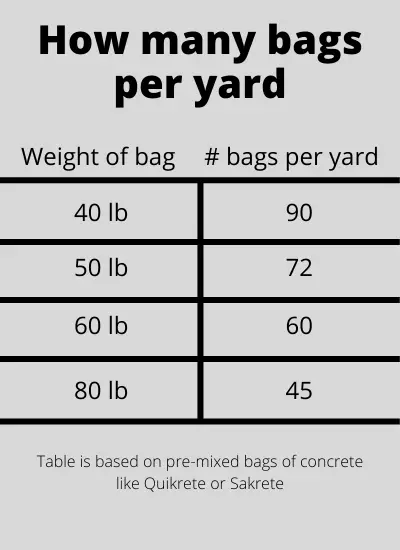## The formula to calculate concrete for a 10 x 10 slab

The formula I use to calculate yards of concrete for a 10' x 10' slab is:

Length x Width x thickness ÷ 27  (all measurements should be in feet)

I'll use a 4 inch thick slab as an example.

First, I calculate the square feet: 10 x 10 = 100 square feet

Second, I calculate the cubic footage by multiplying the square feet by the thickness 100 x .33 = 33 cubic feet. (a 4" thick slab is the same as .33 feet) 4" ÷ 12" = .33 feet

Third, I divide the cubic footage by 27 to get cubic yards (33 ÷ 27 = 1.22 cubic yards)

The number 27 is how many cubic feet are in a cubic yard.  (3x3x3=27)

• For a 3" thick slab use .25 as the thickness
• For a 5" thick slab use .42 as the thickness
• For a 6" thick slab use .5 as the thickness

## how to mix bagged concrete for a 10' x 10' slab

In a wheelbarrow:

Most bags of concrete take about 4 to 5 quarts of water to mix for each bag.

Add the water in the wheelbarrow first, then dump in half the bag and mix.

After half the bag is mixed, add the other half and finish mixing.

You best bet is to use a hoe to mix the bagged concrete in a wheelbarrow.

Make sure to read the mixing instructions on the bag for best results.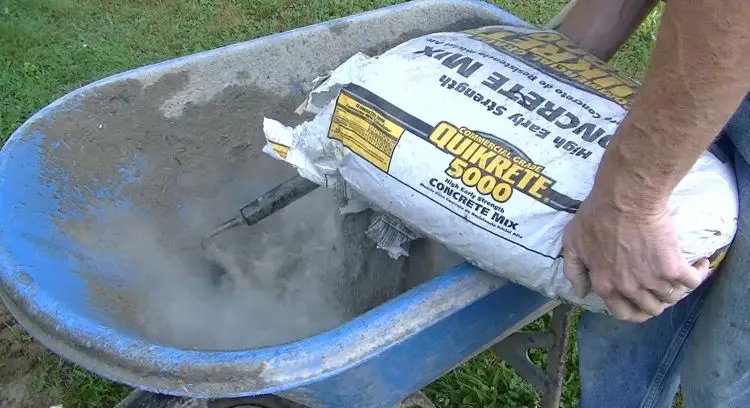In a bucket:

Using a 10 or 20 gallon mixing bucket will work best.

Add the recommended water first and then add half the bag & mix well.

Add the other half and continue mixing for a couple minutes until all the concrete is mixed consistently.

Using an electric mixer drill will make this task much easier.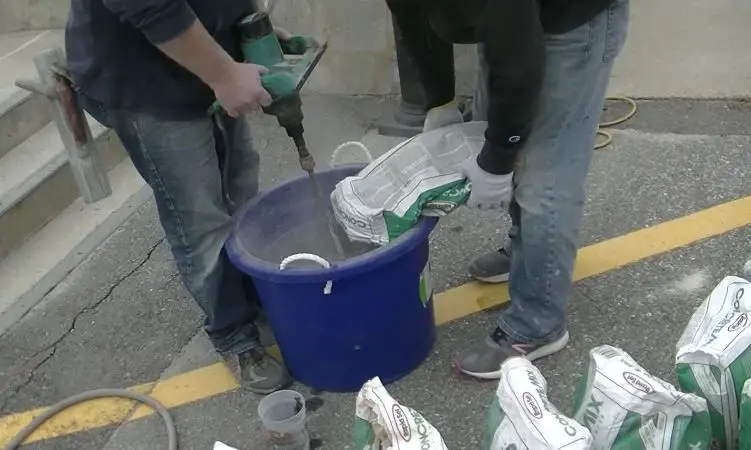In an electric mixer:

You should be able to mix 2 bags at a time if the mixer is large enough.

Add recommended water per bag to the mixer first.

Add 1 bag and mix for a minute, then add the 2nd bag and mix for a couple minutes until the concrete is mixed consistently.

Once mixed, dump the concrete into a wheelbarrow to transport to your slab.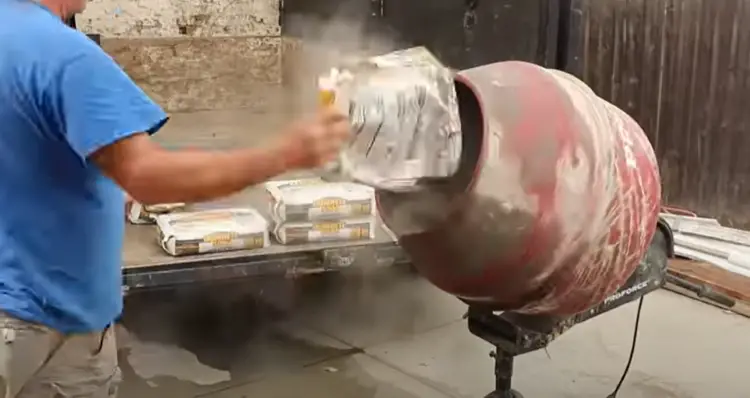## what type of bagged concrete is best for a 10' x 10' slab?

The best type of bag concrete mix to use for a 10x10 slab is 4000 psi or 5000 psi mix.

I like to use Sakrete High Strength Concrete mix which is a 4000 psi mix or I use Sakrete 5000 Plus High Strength Concrete Mix if it's available.

The 5000 psi mix is a little easier to finish because it has more cement paste but both are good.

Both can be purchased in 80 lb. bags